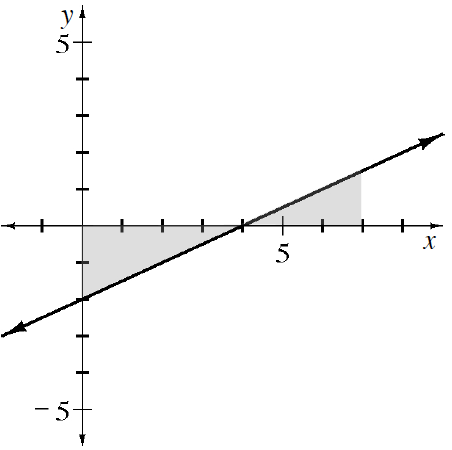### Home > APCALC > Chapter 1 > Lesson 1.2.5 > Problem1-84

1-84.

Examine the graph of $f(x) = 0.5x - 2$ at right.

1. Calculate the area of the shaded region using geometry. (Recall that area below the $x$-axis is considered negative.)

Find the area of each triangle separately. Recall that the area below the $x$-axis is negative. Add the negative and the positive regions together.

$-1\frac{3}{4}$

$\text{[Area of triangle A]} + \text{[Area of triangle B]}$

$\frac{1}{2}(4)(f(0))+\frac{1}{2}(7-4)(f(7))$

$\frac{1}{2}(4)(-2)+\frac{1}{2}(3)(1.5)=$

2. What is the value of $k$ if the area under the curve for $0 ≤ x ≤ k$ is $10$? How did you obtain your solution?

$\text{[Area of triangle A]} + \text{[Area of triangle B]} = 10$

$\frac{1}{2}(4)(f(0))+\frac{1}{2}(k-4)(f(k))=10$

$\frac{1}{2}(4)(f(0))+\frac{1}{2}((0.5x-2)-4)(0.5x-2)=10$

Simplify, and solve for $k$.